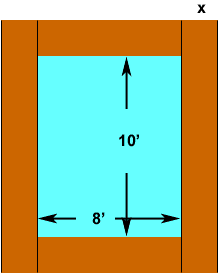Quandaries and Queries Name: Carl Who is asking: Student Level: Secondary Question: A walkway of uniform has 72m2 and surrounds a swimming pool that is 8m wide and 10m long. Find the width of the walkway. Hi Carl, I think you left some words out. I think it should be "A walkway of uniform width has area of 72m2 ..." Did you draw a diagram?Let the width of the walkway be x meters and I drew verticle black lines so that walkway is made up of two short horizontal strips that are each 8 meters by x meters, and two long verticle strips that are each (10+2x) meters by x meters. Hence the area of the walkway is 28x + 2(10 + 2x)x square meters Thus 16 x + 2(10 + 2x)x = 72 Simplify and solve for x. Penny Go to Math Central